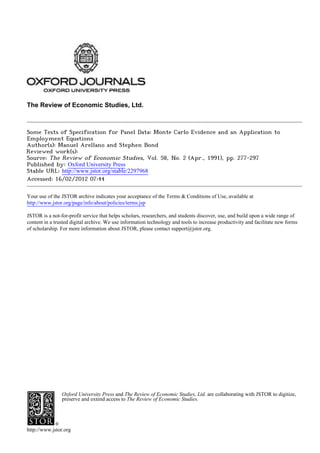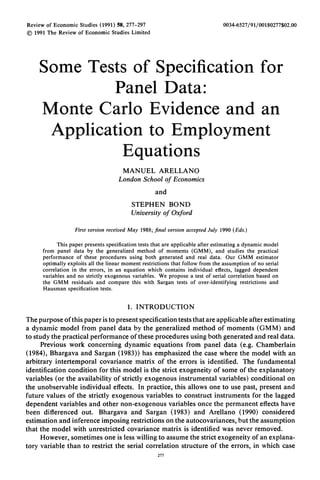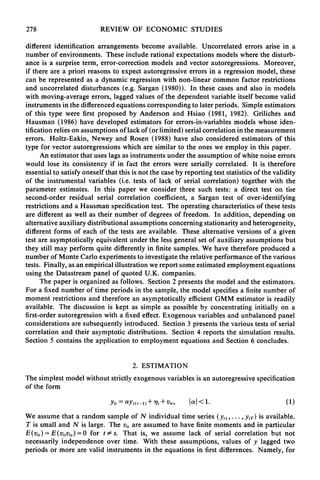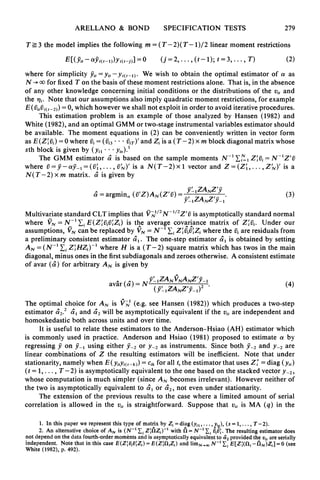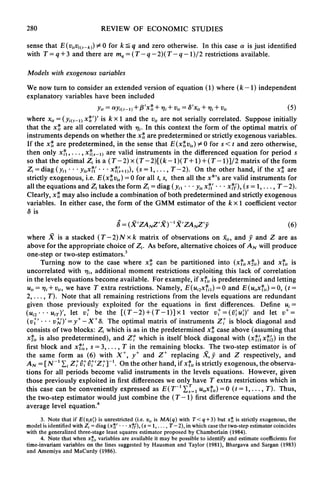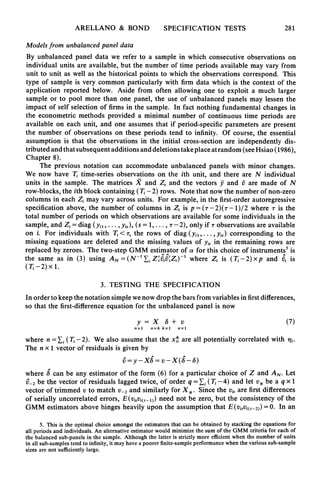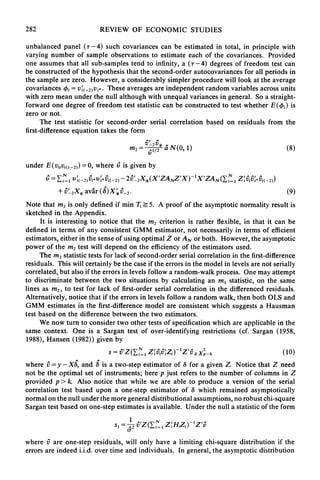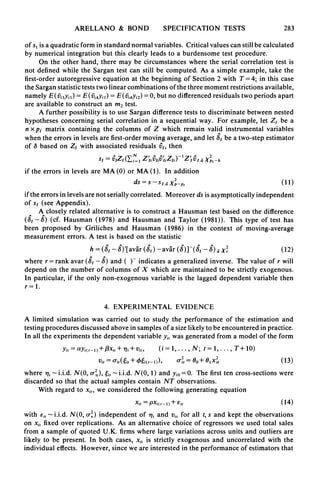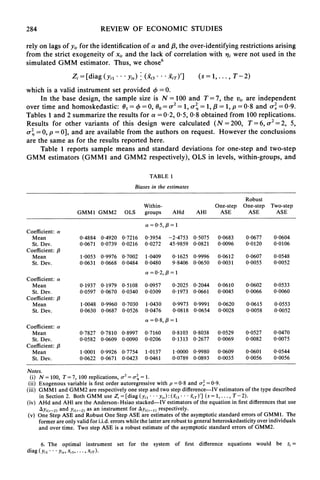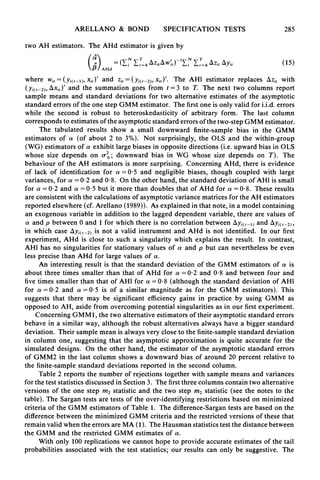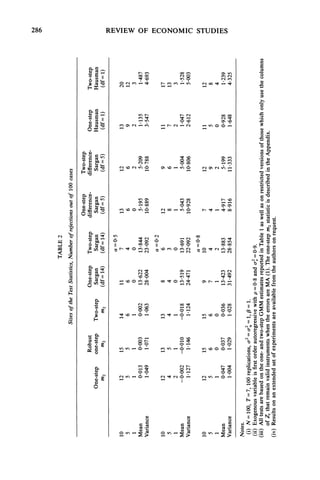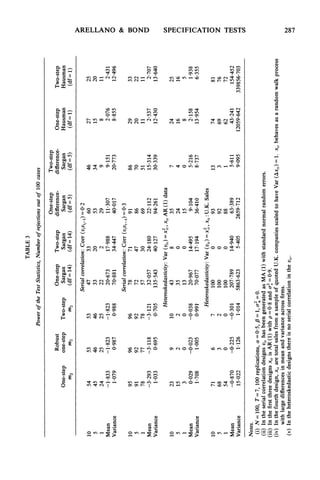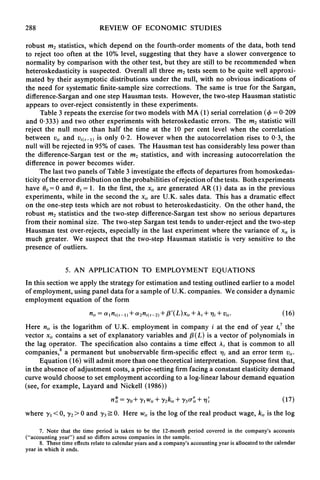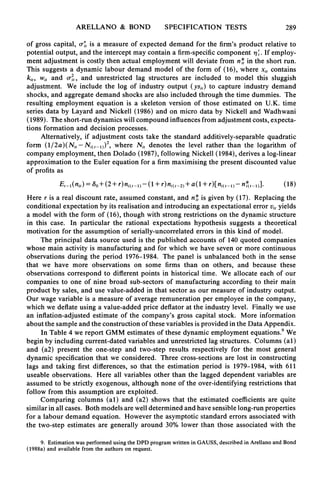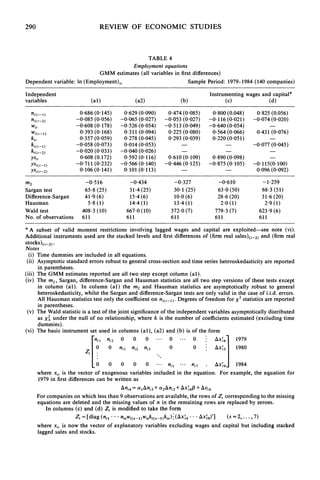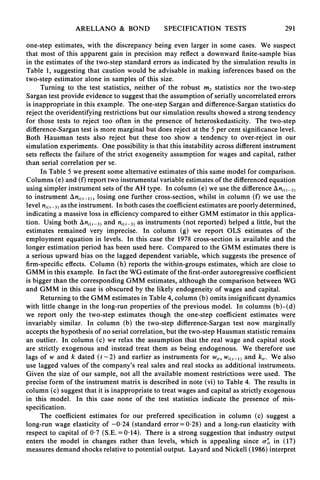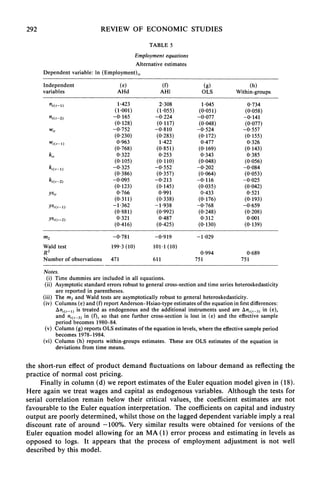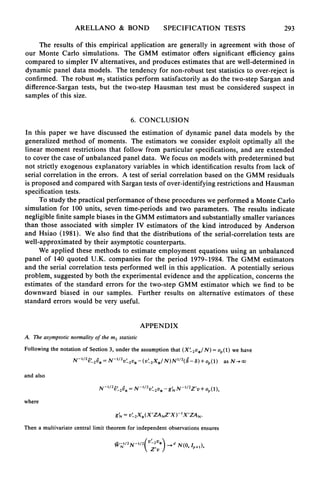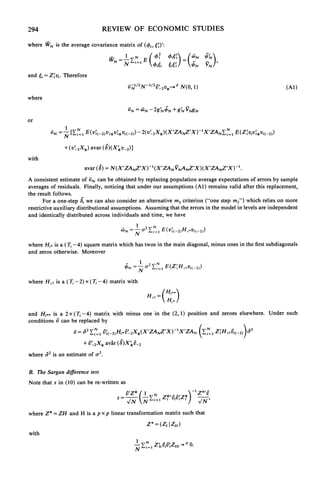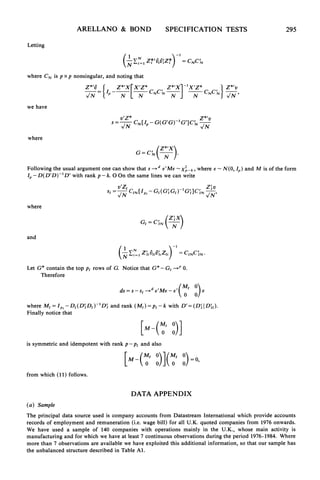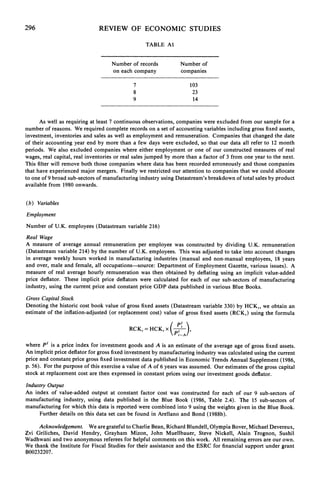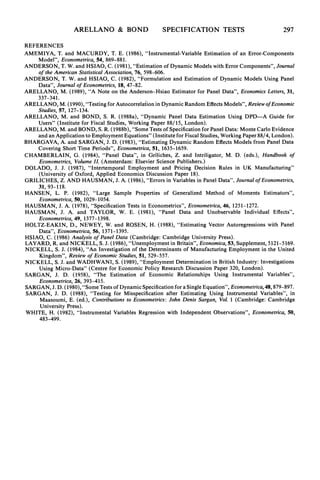Upcoming SlideShare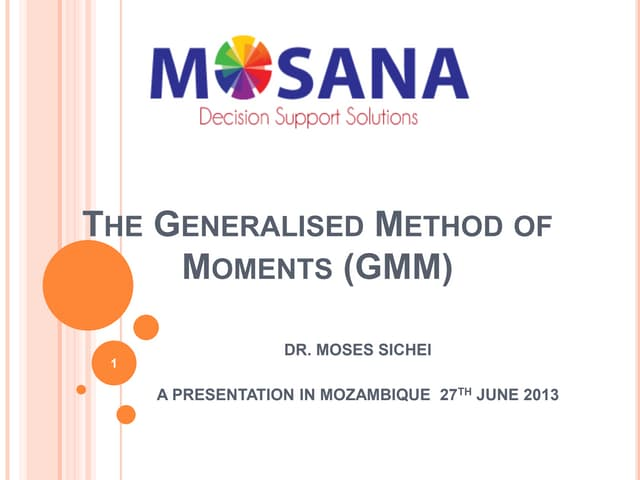Presentation on GMM
1 of 22

### Arellano bond

2. Review of Economic Studies (1991) 58, 277-297 0034-6527/91/00180277\$02.00 ? 1991 The Review of Economic Studies Limited Some Tests of Specification for Panel Data: Monte Carlo Evidence and an Application to Employment Equations MANUEL ARELLANO London School of Economics and STEPHEN BOND Universityof Oxford First version receivedMay 1988;final version acceptedJuly 1990 (Eds.) This paper presents specification tests that are applicable after estimating a dynamic model from panel data by the generalized method of moments (GMM), and studies the practical performance of these procedures using both generated and real data. Our GMM estimator optimally exploits all the linear moment restrictions that follow from the assumption of no serial correlation in the errors, in an equation which contains individual effects, lagged dependent variables and no strictly exogenous variables. We propose a test of serial correlation based on the GMM residuals and compare this with Sargan tests of over-identifying restrictions and Hausman specification tests. 1. INTRODUCTION The purpose of this paper is to presentspecification tests thatareapplicable afterestimating a dynamic model from panel data by the generalized method of moments (GMM) and to study the practical performance of these procedures using both generated and real data. Previous work concerning dynamic equations from panel data (e.g. Chamberlain (1984), Bhargava and Sargan (1983)) has emphasized the case where the model with an arbitrary intertemporal covariance matrix of the errors is identified. The fundamental identification condition for this model is the strict exogeneity of some of the explanatory variables (or the availability of strictly exogenous instrumental variables) conditional on the unobservable individual effects. In practice, this allows one to use past, present and future values of the strictly exogenous variables to construct instruments for the lagged dependent variables and other non-exogenous variables once the permanent effects have been differenced out. Bhargava and Sargan (1983) and Arellano (1990) considered estimation and inference imposing restrictions on the autocovariances, but the assumption that the model with unrestricted covariance matrix is identified was never removed. However, sometimes one is less willing to assume the strictexogeneity of an explana- tory variable than to restrict the serial correlation structure of the errors, in which case 277
3. 278 REVIEW OF ECONOMIC STUDIES different identification arrangements become available. Uncorrelated errors arise in a number of environments. These include rational expectations models where the disturb- ance is a surprise term, error-correction models and vector autoregressions. Moreover, if there are a priori reasons to expect autoregressive errors in a regression model, these can be represented as a dynamic regression with non-linear common factor restrictions and uncorrelated disturbances (e.g. Sargan (1980)). In these cases and also in models with moving-average errors, lagged values of the dependent variable itself become valid instruments in the differenced equations corresponding to later periods. Simple estimators of this type were first proposed by Anderson and Hsiao (1981, 1982). Griliches and Hausman (1986) have developed estimators for errors-in-variables models whose iden- tification relies on assumptions of lack of (or limited) serial correlation in the measurement errors. Holtz-Eakin, Newey and Rosen (1988) have also considered estimators of this type for vector autoregressions which are similar to the ones we employ in this paper. An estimator that uses lags as instrumentsunder the assumption of white noise errors would lose its consistency if in fact the errors were serially correlated. It is therefore essential to satisfy oneself that this is not the case by reporting test statistics of the validity of the instrumental variables (i.e. tests of lack of serial correlation) together with the parameter estimates. In this paper we consider three such tests: a direct test on tne second-order residual serial correlation coefficient, a Sargan test of over-identifying restrictions and a Hausman specification test. The operating characteristics of these tests are different as well as their number of degrees of freedom. In addition, depending on alternative auxiliary distributional assumptions concerning stationarityand heterogeneity, different forms of each of the tests are available. These alternative versions of a given test are asymptotically equivalent under the less general set of auxiliary assumptions but they still may perform quite differently in finite samples. We have therefore produced a number of Monte Carlo experiments to investigate the relative performance of the various tests. Finally, as an empirical illustration we reportsome estimated employment equations using the Datastream panel of quoted U.K. companies. The paper is organized as follows. Section 2 presents the model and the estimators. For a fixed number of time periods in the sample, the model specifies a finite number of moment restrictions and therefore an asymptotically efficient GMM estimator is readily available. The discussion is kept as simple as possible by concentrating initially on a first-orderautoregression with a fixed effect. Exogenous variables and unbalanced panel considerations are subsequently introduced. Section 3 presents the various tests of serial correlation and their asymptotic distributions. Section 4 reports the simulation results. Section 5 contains the application to employment equations and Section 6 concludes. 2. ESTIMATION The simplest model without strictly exogenous variables is an autoregressive specification of the form Yi,= ayi(t-l)++,i + Vit, lal < 1. (1) We assume that a random sample of N individual time series (yi,... ., YiT) is available. T is small and N is large. The vi, are assumed to have finite moments and in particular E(vit) = E(vitvij) =0 for t \$ s. That is, we assume lack of serial correlation but not necessarily independence over time. With these assumptions, values of y lagged two periods or more are valid instruments in the equations in first differences. Namely, for
4. ARELLANO & BOND SPECIFICATION TESTS 279 Ti_3 the model implies the following m=(T-2)(T-1)/2 linear moment restrictions E[(yit-aY-(t_))yj(t_j) = 0 (j = 2, ... , (t -1); t = 3, .. ., T) (2) where for simplicity Yi = Yi- Yi(t-i) We wish to obtain the optimal estimator of a as N - cofor fixed T on the basis of these moment restrictions alone. That is, in the absence of any other knowledge concerning initial conditions or the distributions of the vi, and the qj. Note that our assumptions also imply quadratic moment restrictions, for example E( eit5i(t-2))= 0, which however we shall not exploit in orderto avoid iterative procedures. This estimation problem is an example of those analyzed by Hansen (1982) and White (1982), and an optimal GMM or two-stage instrumental variables estimator should be available. The moment equations in (2) can be conveniently written in vector form as E(Z'ibi) = 0 where vi = (vi3 . .. lbiT)'and Zi is a (T-2) x m block diagonal matrixwhose sth block is given by (yi *... Yis) The GMM estimator a' is based on the sample moments N-'1 = Z'i3 = N-'Z'i where v3=y-ajLl=(iU',..., I'N)' is a N( T-2)x1 vector and Z=(Z,..., Z'N)'is a N(T - 2) x m matrix. a is given by a = argmina (ViZ)AN(Z') = -lZANZ (3) Y_,ZANZ'Y-l' Multivariate standard CLT implies that V-1P2N-"Z2Z'I is asymptotically standardnormal where VN= N- 'E E(Z'ifAiZj) is the average covariance matrix of Ziui. Under our assumptions, VNcan be replaced by VN= N Zivi3Z, where theIi are residuals from a preliminary consistent estimator at. The one-step estimator ca is obtained by setting AN = (N-1 Ei ZHZi)-l where H is a (T-2) square matrix which has twos in the main diagonal, minus ones in the firstsubdiagonals and zeroes otherwise. A consistent estimate of avar (c) for arbitraryAN is given by aa (a) =NY lZANVNANZ'Y-1avar( a=N - ZN,p_) 4 The optimal choice for AN is VN1(e.g. see Hansen (1982)) which produces a two-step A 2 A A estimator a2. a, and a2 will be asymptotically equivalent if the vi, are independent and homoskedastic both across units and over time. It is useful to relate these estimators to the Anderson-Hsiao (AH) estimator which is commonly used in practice. Anderson and Hsiao (1981) proposed to estimate a by regressing y on Y-1 using either Y-2 or Y-2 as instruments. Since both Y-2 and Y-2 are linear combinations of Z the resulting estimators will be inefficient. Note that under stationarity, namely when E(YitYi(t-k)) = Cik for all t,the estimator that uses Z+ = diag (yit) (t = 1, .. ., T - 2) is asymptotically equivalent to the one based on the stacked vector Y-2, whose computation is much simpler (since AN becomes irrelevant). However neither of the two is asymptotically equivalent to a, or a2, not even under stationarity. The extension of the previous results to the case where a limited amount of serial correlation is allowed in the vit is straightforward. Suppose that vit is MA (q) in the 1. In this paper we represent this type of matrix by Zi = diag (yil,. Yis), (s = 1 ... i T-2). 2. An alternative choice of AN is (N- 1 ZfAlZi)-l with A = N-1 S The resulting estimator does not depend on the data fourth-order moments and is asymptotically equivalent to '2 provided the vi, are serially independent. Note that in this case E(Z!ti3ivZi) = E(Z!fliZi) and limN o0 N-1 , E[Z!(fli -fN)Zi] = O(see White (1982), p. 492).
5. 280 REVIEW OF ECONOMIC STUDIES sense that E(vivi(,_k)) \$ Ofor k _ q and zero otherwise. In this case a is just identified with T = q+ 3 and there are mq T- q- 2)( T - q- 1)/2 restrictions available. Models with exogenous variables We now turn to consider an extended version of equation (1) where (k -1) independent explanatory variables have been included Yit= ayi(t,l)+ xU*+ 'qi+ Vit= 8'xi, + 7Ri+ vi, (5) where xit= (Yi(t-1) x*')' is k x 1 and the vit are not serially correlated. Suppose initially that the x* are all correlated with qi. In this context the form of the optimal matrix of instruments depends on whether the x* are predetermined or strictly exogenous variables. If the x* are predetermined, in the sense that E(xi*vis) \$ 0 for s < t and zero otherwise, then only x*, ... , x*4s-1) are valid instruments in the differenced equation for period s so that the optimal Zi is a (T-2)x(T-2)[(k-1)(T+1)+(T-1)]/2 matrix of the form Zi = diag (yi, ... yisx*l ... x*( +1)), (s = 1, . . ., T - 2). On the other hand, if the x* are strictly exogenous, i.e. E(x viis) = 0 for all t, s, then all the x*'s are valid instruments for all the equations and Zi takes the form Zi = diag (yi, * yisx*x'* X** ), (s = 1,..., T- 2). Clearly, x* may also include a combination of both predetermined and strictlyexogenous variables. In either case, the form of the GMM estimator of the k x 1 coefficient vector 8 is = (X'ZANZ'X) X'ZANZ'y (6) where X is a stacked (T -2) N x k matrix of observations on xit, and y and Z are as above for the appropriate choice of Zi. As before, alternative choices of AN will produce one-step or two-step estimators.3 Turning now to the case where x* can be partitioned into (x*t,x*x,) and x*, is uncorrelated with qi, additional moment restrictions exploiting this lack of correlation in the levels equations become available. For example, if x*, is predetermined and letting uit= qi+ vi,, we have T extra restrictions. Namely, E(ui2XIi4) = 0 and E (ui,xI,i)= 0, (t = 2,..., T). Note that all remaining restrictions from the levels equations are redundant given those previously exploited for the equations in first differences. Define ui= (Ui2 . . UiTY), let v+ be the [(T-2)+(T-1)]xl vector v+=(i3u')' and let v+= (V1+' ... v+' Y+- X+8. The optimalmatrixof instrumentsZ+ is block diagonaland consists of two blocks: Zi which is as in the predetermined x* case above (assuming that x* t is also predetermined), and Za which is itself block diagonal with (x*lx*42) in the first block and x*', s = 3, .. ., T in the remaining blocks. The two-step estimator is of the same form as (6) with X+, y+ and Z+ replacing X, y and Z respectively, and AN = [N-1 Eji Z+A+A+t'ZP+]-. On the other hand, if x4*tis strictly exogenous, the observa- tions for all periods become valid instruments in the levels equations. However, given those previously exploited in first differences we only have T extra restrictions which in this case can be conveniently expressed as E(T-1 ,i=1uisxit) = 0 (t= 1,..., T). Thus, the two-step estimator would just combine the (T- 1) first difference equations and the average level equation.4 3. Note that if E(vjvt) is unrestricted (i.e. vi, is MA(q) with T< q+3) but x* is strictly exogenous, the modelisidentifiedwithZ, = diag(* ... **x), (s = 1, . . ., T- 2), in which case the two-step estimatorcoincides with the generalized three-stage least squares estimator proposed by Chamberlain (1984). 4. Note that when x*, variables are available it may be possible to identify and estimate coefficients for time-invariant variables on the lines suggested by Hausman and Taylor (1981), Bhargava and Sargan (1983) and Amemiya and MaCurdy (1986).
6. ARELLANO & BOND SPECIFICATION TESTS 281 Models from unbalancedpanel data By unbalanced panel data we refer to a sample in which consecutive observations on individual units are available, but the number of time periods available may vary from unit to unit as well as the historical points to which the observations correspond. This type of sample is very common particularly with firm data which is the context of the application reported below. Aside from often allowing one to exploit a much larger sample or to pool more than one panel, the use of unbalanced panels may lessen the impact of self selection of firms in the sample. In fact nothing fundamental changes in the econometric methods provided a minimal number of continuous time periods are available on each unit, and one assumes that if period-specific parameters are present the number of observations on these periods tend to infinity. Of course, the essential assumption is that the observations in the initial cross-section are independently dis- tributedandthatsubsequent additions anddeletions takeplace atrandom(see Hsiao (1986), Chapter 8). The previous notation can accommodate unbalanced panels with minor changes. We now have Ti time-series observations on the ith unit, and there are N individual units in the sample. The matrices X and Z, and the vectors y- and v are made of N row-blocks, the ith block containing (Ti - 2) rows. Note that now the number of non-zero columns in each Zi may vary across units. For example, in the first-orderautoregressive specification above, the number of columns in Zi is p = (-r- 2)(-r- 1)/2 where r is the total number of periods on which observations are available for some individuals in the sample, and Zi = diag (yi , .. , Yis),(s = 1, . . ., r-2), only if r observations are available on i. For individuals with Ti< r, the rows of diag (yi,.... , yis) corresponding to the missing equations are deleted and the missing values of Yitin the remaining rows are replaced by zeroes. The two-step GMM estimator of a for this choice of instruments: is the same as in (3) using AN=(N-'E'Z'ivZiZl)-1 where Zi is (Ti-2)xp and vi is (Ti -2) x 1. 3. TESTING THE SPECIFICATION In orderto keep the notation simple we now drop the barsfromvariables in firstdifferences, so that the first-difference equation for the unbalanced panel is now y=X 8+ v (7) nxl nxk kxl nxl where n = i (Ti -2). We also assume that the x* are all potentially correlated with m1i. The n x 1 vector of residuals is given by A=y-X8= v-X(8-8) where 8 can be any estimator of the form (6) for a particular choice of Z and AN. Let v2 be the vector of residuals lagged twice, of order q = i (1Ti- 4) and let v* be a q x 1 vector of trimmed v to match v-2 and similarly for X*. Since the vitare first differences of serially uncorrelated errors, E(vitvi(t-l)) need not be zero, but the consistency of the GMM estimators above hinges heavily upon the assumption that E(vitvi(t-2)) =0. In an 5. This is the optimal choice amongst the estimators that can be obtained by stacking the equations for all periods and individuals. An alternative estimator would minimize the sum of the GMM criteria for each of the balanced sub-panels in the sample. Although the latter is strictly more efficient when the number of units in all sub-samples tend to infinity, it may have a poorer finite-sample performance when the various sub-sample sizes are not sufficiently large.
7. 282 REVIEW OF ECONOMIC STUDIES unbalanced panel (r-4) such covariances can be estimated in total, in principle with varying number of sample observations to estimate each of the covariances. Provided one assumes that all sub-samples tend to infinity, a (r-4) degrees of freedom test can be constructed of the hypothesis that the second-order autocovariances for all periods in the sample are zero. However, a considerably simpler procedure will look at the average covariances Xi- (2)V These averages are independent random variables across units with zero mean under the null although with unequal variances in general. So a straight- forward one degree of freedom test statistic can be constructed to test whether E(4i) is zero or not. The test statistic for second-order serial correlation based on residuals from the first-difference equation takes the form M2 '-A1/2* dIN(0, 1) (8) V under E(vitvi(t-2)) =0, where v is given by V = Z,1-1 V 2) Vi* V* V'i(-2)- 2 '2X*(X'ZANZXY)XPZAN(N Zviv i*Vi(-2)) + v' 2X avar (8)X *V2. (9) Note that m2is only defined if min Ti-' 5. A proof of the asymptotic normality result is sketched in the Appendix. It is interesting to notice that the m2 criterion is rather flexible, in that it can be defined in terms of any consistent GMM estimator, not necessarily in terms of efficient estimators, either in the sense of using optimal Z or AN or both. However, the asymptotic power of the m2test will depend on the efficiency of the estimators used. The m2statistic tests for lack of second-order serial correlation in the first-difference residuals. This will certainly be the case if the errorsin the model in levels are not serially correlated, but also if the errorsin levels follow a random-walk process. One may attempt to discriminate between the two situations by calculating an ml statistic, on the same lines as M2, to test for lack of first-order serial correlation in the differenced residuals. Alternatively, notice that if the errorsin levels follow a random walk, then both OLS and GMM estimates in the first-difference model are consistent which suggests a Hausman test based on the difference between the two estimators. We now turn to consider two other tests of specification which are applicable in the same context. One is a Sargan test of over-identifying restrictions (cf. Sargan (1958, 1988), Hansen (1982)) given by SV=Z(Zl1 ZiviZZi) YZVa Xp-k (10) where v = y - X8, and 8 is a two-step estimator of 8 for a given Z. Notice that Z need not be the optimal set of instruments; here p just refers to the number of columns in Z provided p > k. Also notice that while we are able to produce a version of the serial correlation test based upon a one-step estimator of 8 which remained asymptotically normal on the null underthe more general distributional assumptions, no robustchi-square Sargantest based on one-step estimates is available. Under the null a statistic of the form l=A2V Z(E-I ZHiHZi)- Z=V where v-are one-step residuals, will only have a limiting chi-square distribution if the errorsare indeed i.i.d. over time and individuals. In general, the asymptotic distribution
8. ARELLANO & BOND SPECIFICATION TESTS 283 of s1is a quadraticform in standardnormalvariables. Criticalvalues can still be calculated by numerical integration but this clearly leads to a burdensome test procedure. On the other hand, there may be circumstances where the serial correlation test is not defined while the Sargan test can still be computed. As a simple example, take the first-orderautoregressive equation at the beginning of Section 2 with T= 4; in this case the Sarganstatistictests two linear combinations of the three moment restrictionsavailable, namely E(1Ui3yi1)= E(lbi4yi1) = E(ti4yi2) = 0, but no differenced residuals two periods apart are available to construct an m2test. A further possibility is to use Sargan difference tests to discriminate between nested hypotheses concerning serial correlation in a sequential way. For example, let Z, be a n x p, matrix containing the columns of Z which remain valid instrumental variables when the errorsin levels are first-ordermoving average, and let 8, be a two-step estimator of 8 based on Z, with associated residuals v^,then S1 = VIZ,(-1 I viiIi IV Ia -k if the errors in levels are MA (0) or MA (1). In addition ds= s-_SIaX'2-P0 1l) if the errorsin levels arenot serially correlated. Moreover ds is asymptotically independent of s, (see Appendix). A closely related alternative is to construct a Hausman test based on the difference (8, -8) (cf. Hausman (1978) and Hausman and Taylor (1981)). This type of test has been proposed by Griliches and Hausman (1986) in the context of moving-average measurement errors. A test is based on the statistic h = ,(? - )'[av'r (?SI)- ava'r(3)]-(?s- ?)a x2(12) A A where r= rank avar (3, -8) and ( ) indicates a generalized inverse. The value of r will depend on the number of columns of X which are maintained to be strictly exogenous. In particular, if the only non-exogenous variable is the lagged dependent variable then r=1. 4. EXPERIMENTAL EVIDENCE A limited simulation was carried out to study the performance of the estimation and testing procedures discussed above in samples of a size likely to be encountered in practice. In all the experiments the dependent variable yi, was generated from a model of the form Yit= aYi(,-I)+ oxit ,q7i+vi, (i= 19 ... ., N; t= 1, ...., T+ 10) Vit = 07it(eit + 4ei(t-)), it = 00+ 1Xit (13) where q,i i.i.d. N(O, o-2 ), {it - i.i.d. N(0, 1) and yio= 0. The firstten cross-sections were discarded so that the actual samples contain NT observations. With regard to xi,, we considered the following generating equation xit = pxi(,-,) + 6it (14) with Eit-i.i.d. N(O, (J2) independent of qi and vi, for all t, s and kept the observations on xi, fixed over replications. As an alternative choice of regressors we used total sales from a sample of quoted U.K. firms where large variations across units and outliers are likely to be present. In both cases, xi, is strictly exogenous and uncorrelated with the individual effects. However, since we are interested in the performance of estimators that
9. 284 REVIEW OF ECONOMIC STUDIES rely on lags of yi, for the identification of a and ,3,the over-identifying restrictions arising from the strict exogeneity of xi, and the lack of correlation with -qiwere not used in the simulated GMM estimator. Thus, we chose6 Zi- [diag(yi, ..**Yis)*C(X3 ** iTY ] (S = 1I. . T-2) which is a valid instrument set provided 4?=0. In the base design, the sample size is N = 100 and T =7, the vi, are independent over time and homoskedastic: 0, =4?= 0, 00= a.2 = 1 (o2 = 1, / = 1, p = 0-8 and or2 = 0-9. Tables 1 and 2 summarize the results for a = 0-2, 0 5, 0 8 obtained from 100 replications. Results for other variants of this design were calculated (N = 200, T =6, r.2=2, 5, 2 = 0, p = 0], and are available from the authors on request. However the conclusions are the same as for the results reported here. Table 1 reports sample means and standard deviations for one-step and two-step GMM estimators (GMM1 and GMM2 respectively), OLS in levels, within-groups, and TABLE 1 Biases in the estimates Robust Within- One-step One-step Two-step GMM1 GMM2 OLS groups AHd AHI ASE ASE ASE a =0 5, , =1 Coefficient: a Mean 0-4884 0-4920 0-7216 0 3954 -2-4753 0 5075 0-0683 0-0677 0-0604 St. Dev. 0-0671 0 0739 0-0216 0-0272 45-9859 0-0821 0-0096 0-0120 0-0106 Coefficient: ,3 Mean 1-0053 0-9976 0-7002 1-0409 0-1625 0-9996 0-0612 0-0607 0-0548 St. Dev. 0-0631 0-0668 0-0484 0-0480 9-8406 0-0650 0-0031 0-0055 0-0052 a = 0-2, ,3= 1 Coefficient: a Mean 0-1937 0d1979 0-5108 0-0957 0-2025 0-2044 0-0610 0-0602 0-0533 St. Dev. 0 0597 0-0670 0 0340 0 0309 0-1973 0-0661 0 0045 0-0066 0-0060 Coefficient: /8 Mean 1-0048 0-9960 0 7030 1-0430 0-9973 0-9991 0-0620 0-0615 0-0553 St. Dev. 0-0630 0-0687 0-0526 0-0476 0-0818 0-0654 0-0028 0-0058 0-0052 a =0-8,/3 = 1 Coefficient: a Mean 0-7827 0-7810 0-8997 0-7160 0-8103 0-8038 0-0529 0-0527 0 0470 St. Dev. 0-0582 0-0609 0 0090 0-0206 0-1313 0-2677 0-0069 0-0082 0 0075 Coefficient: ,3 Mean 1-0001 0-9926 0-7754 1-0137 1-0000 0-9980 0-0609 0-0601 0-0544 St. Dev. 0-0622 0-0671 0-0423 0-0461 0-0789 0-0893 0 0035 0-0056 0-0056 Notes. (i) N = 100, T= 7, 100 replications, o-2= a2 1. (ii) Exogenous variable is first order autoregressive with p = 0-8 and or = 0 9. (iii) GMMI and GMM2 are respectively one step and two step difference-IV estimators of the type described in Section 2. Both GMM use Z, = [diag (y,, ... Yi) (Xj3 * * * ,T)'] (S = 1, .* *, T-2). (iv) AHd and AHl are the Anderson-Hsiao stacked-IV estimators of the equation in firstdifferences that use AYi(,-2) and Yi(t-2) as an instrument for Ayi(,-1) respectively. (v) One Step ASE and Robust One Step ASE are estimates of the asymptotic standard errorsof GMM1. The former are only valid for i.i.d. errorswhile the latterare robustto general heteroskedasticity over individuals and over time. Two step ASE is a robust estimate of the asymptotic standard errors of GMM2. 6. The optimal instrument set for the system of first difference equations would be zi= diag (yi, *.* Yis, Xi, - -, XiT)-
10. ARELLANO & BOND SPECIFICATION TESTS 285 two AH estimators. The AHd estimator is given by ( ^) = (i Et=4 AZitw I)t t 4 AZit Ayit (15) where wi = (yi(t_1), xit)' and zi, = (yi(t-2), xi,)'. The AHI estimator replaces Azi, with (yi(t-2), Axit)' and the summation goes from t =3 to T The next two columns report sample means and standard deviations for two alternative estimates of the asymptotic standard errorsof the one step GMM estimator. The firstone is only valid for i.i.d. errors while the second is robust to heteroskedasticity of arbitrary form. The last column corresponds to estimates of the asymptotic standarderrorsof the two-step GMM estimator. The tabulated results show a small downward finite-sample bias in the GMM estimators of a (of about 2 to 3%). Not surprisingly, the OLS and the within-group (WG) estimators of a exhibit large biases in opposite directions (i.e. upward bias in OLS whose size depends on (J2; downward bias in WG whose size depends on T). The behaviour of the AH estimators is more surprising. Concerning AHd, there is evidence of lack of identification for a = 05 and negligible biases, though coupled with large variances, for a-= 0-2 and 0-8. On the other hand, the standard deviation of AHl is small for a = 0-2 and a = 0 5 but it more than doubles that of AHd for a = 0-8. These results are consistent with the calculations of asymptotic variance matrices for the AH estimators reported elsewhere (cf. Arellano (1989)). As explained in that note, in a model containing an exogenous variable in addition to the lagged dependent variable, there are values of a and p between 0 and 1 for which there is no correlation between AYi(,t-) and AYi(,-2), in which case AYi(t-2) is not a valid instrument and AHd is not identified. In our first experiment, AHd is close to such a singularity which explains the result. In contrast, AHl has no singularities for stationary values of a and p but can nevertheless be even less precise than AHd for large values of a. An interesting result is that the standard deviation of the GMM estimators of a is about three times smaller than that of AHd for a = 0-2 and 0-8 and between four and five times smaller than that of AHl for a = 0-8 (although the standard deviation of AHl for a = 0-2 and a =0 -5 is of a similar magnitude as for the GMM estimators). This suggests that there may be significant efficiency gains in practice by using GMM as opposed to AH, aside from overcoming potential singularities as in our firstexperiment. Concerning GMM1, the two alternative estimators of their asymptotic standard errors behave in a similar way, although the robust alternatives always have a bigger standard deviation. Their sample mean is always very close to the finite-sample standard deviation in column one, suggesting that the asymptotic approximation is quite accurate for the simulated designs. On the other hand, the estimator of the asymptotic standard errors of GMM2 in the last column shows a downward bias of around 20 percent relative to the finite-sample standard deviations reported in the second column. Table 2 reports the number of rejections together with sample means and variances for the test statistics discussed in Section 3. The firstthree columns contain two alternative versions of the one step m2 statistic and the two step m2 statistic (see the notes to the table). The Sargan tests are tests of the over-identifying restrictions based on minimized criteria of the GMM estimators of Table 1. The difference-Sargan tests are based on the difference between the minimized GMM criteria and the restricted versions of these that remainvalid when the errorsare MA (1). The Hausman statisticstest the distance between the GMM and the restricted GMM estimates of a. With only 100 replications we cannot hope to provide accurate estimates of the tail probabilities associated with the test statistics; our results can only be suggestive. The
11. 286 REVIEW OF ECONOMIC STUDIES TABLE 2 Sizes of the Test Statistics, Number of rejections out of 100 cases One-step Two-step Robust One-step Two-step difference- difference- One-step Two-step One-step one-step Two-step Sargan Sargan Sargan Sargan Hausman Hausman M2 M2 M2 (df= 14) (df= 14) (df= 5) (df= 5) (df= 1) (df= 1) a=0-5 10 12 15 14 11 7 13 12 13 20 5 5 5 6 6 4 6 6 9 12 1 1 1 1 0 0 0 2 2 3 Mean 0-013 0-003 0-002 13-622 13-844 5-195 5-209 1-135 1-487 Variance 1-049 1-071 1-063 28-004 23-092 10-889 10-788 3-547 4-693 a = 0-2 10 12 13 13 8 6 12 9 11 17 5 4 5 4 4 3 8 6 7 13 1 2 1 1 0 0 1 1 2 3 Mean -0-002 -0-010 -0-018 13-519 13-691 5 043 5 004 1-047 1-528 Variance 1-127 1-146 1-124 24-471 22-092 10-928 10-806 2-612 5 003 a = 0-8 10 12 15 15 9 10 7 12 11 12 5 5 6 6 7 4 4 9 5 8 1 1 0 0 1 2 1 2 0 4 Mean 0 047 0 037 0-036 13-423 13-883 4-917 5-199 0-928 1-239 Variance 1-004 1-029 1-028 31-492 28-854 8-916 11-333 1-648 4-325 Notes. (i) N = 100, T = 7, 100 replications, U2 = U= 1 p = 1. (ii) Exogenous variable is first order autoregressive with p = 0-8 and o2 = 09- (iii) All tests are based on the one- and two-step GMM estimates reported in Table 1 as well as on restricted versions of those which only use the columns of Zi that remain valid instruments when the errors are MA (1). The one-step m2 statistic is described in the Appendix. (iv) Results on an extended set of experiments are available from the authors on request.
12. ARELLANO & BOND SPECIFICATION TESTS 287 TABLE 3 Power of the Test Statistics, Number of rejections out of 100 cases One-step Two-step Robust One-step Two-step difference- difference- One-step Two-step One-step one-step Two-step Sargan Sargan Sargan Sargan Hausman Hausman M2 M2 M2 (df= 14) (df= 14) (df= 5) (df= 5) (df= 1) (df= 1) Serial correlation: Corr (v,v,1) = 0 2 10 54 53 53 47 33 60 46 27 25 5 45 46 46 33 20 53 34 15 20 1 24 25 25 22 2 29 9 8 11 Mean -1-833 -1-823 -1-823 20-673 17-988 11-307 9-151 2-076 2-431 Variance 1-079 0-987 0-988 70-881 34-447 40-017 20-773 8-855 12-496 Serial correlation: Corr (v,v,l) = 0 3 10 95 96 96 78 71 91 86 29 33 5 91 92 92 72 47 86 70 20 22 1 78 77 78 57 30 69 51 11 11 Mean -3-293 -3-118 -3-121 32-057 24-180 22-112 15*314 2-537 2-707 Variance 1-033 0-695 0 705 135-543 40-127 94-261 30 339 12-430 13-640 Heteroskedasticity: Var (vi,) =xi, , Xit AR (1) data 10 23 9 10 43 8 35 7 24 25 5 15 2 2 35 0 24 4 16 16 1 3 0 0 13 0 15 0 8 5 Mean 0-029 -0-023 -0-038 20-967 14-495 9 104 5-216 2-158 1-938 Variance 1-708 1-005 0-991 70 077 17-194 36-410 8-737 13-954 6-355 Heteroskedasticity: Var (Vi,) = xi,, : U.K. Sales 10 71 6 7 100 0 93 13 74 81 5 68 3 2 100 0 92 3 69 76 1 54 0 0 100 0 88 1 62 72 Mean -0-870 -0-225 -0-301 207-789 14-940 63-389 5*611 43-241 154-452 Variance 15-022 1-126 1-014 5863-623 5 405 2659-712 9 095 12059*642 339856*703 Notes. (i) N = 100, T 7, 100 replications, a = 0 5, /3 = 1, o2 = 0. (ii) In the serial correlation designs vi, has been generated as MA (1) with standard normal random errors. (iii) In the first three designs xi, is AR (1) with p = 0-8 and T. = 09. (iv) In the fourth design, xi, are total sales from a sample of quoted U.K. companies scaled to have Var (Axi,) = 1. x,, behaves as a random walk process with large differences in mean and variance across firms. (v) In the heteroskedastic designs there is no serial correlation in the vi,.
13. 288 REVIEW OF ECONOMIC STUDIES robust m2 statistics, which depend on the fourth-order moments of the data, both tend to reject too often at the 10%level, suggesting that they have a slower convergence to normality by comparison with the other test, but they are still to be recommended when heteroskedasticity is suspected. Overall all three m2tests seem to be quite well approxi- mated by their asymptotic distributions under the null, with no obvious indications of the need for systematic finite-sample size corrections. The same is true for the Sargan, difference-Sargan and one step Hausman tests. However, the two-step Hausman statistic appears to over-reject consistently in these experiments. Table 3 repeats the exercise for two models with MA (1) serial correlation (4 = 0209 and 0.333) and two other experiments with heteroskedastic errors. The m2statistic will reject the null more than half the time at the 10 per cent level when the correlation between vi, and vi(,-i) is only 02. However when the autocorrelation rises to 03, the null will be rejected in 95%of cases. The Hausman test has considerably less power than the difference-Sargan test or the m2 statistics, and with increasing autocorrelation the difference in power becomes wider. The last two panels of Table 3 investigate the effects of departuresfrom homoskedas- ticity of the errordistribution on the probabilities of rejection of the tests. Both experiments have 00= 0 and 01= 1. In the first, the xi, are generated AR (1) data as in the previous experiments, while in the second the xi, are U.K. sales data. This has a dramatic effect on the one-step tests which are not robust to heteroskedasticity. On the other hand, the robust m2 statistics and the two-step difference-Sargan test show no serious departures from their nominal size. The two-step Sargan test tends to under-reject and the two-step Hausman test over-rejects, especially in the last experiment where the variance of xi, is much greater. We suspect that the two-step Hausman statistic is very sensitive to the presence of outliers. 5. AN APPLICATION TO EMPLOYMENT EQUATIONS In this section we apply the strategy for estimation and testing outlined earlierto a model of employment, using panel data for a sample of U.K. companies. We consider a dynamic employment equation of the form nit= a, ni(t-l)+ a2ni(t-2) + i'(L)xit + At+ qi+ vit. (16) Here ni, is the logarithm of U.K. employment in company i at the end of year t,7 the vector xi, contains a set of explanatory variables and 13(L) is a vector of polynomials in the lag operator. The specification also contains a time effect Atthat is common to all companies,8 a permanent but unobservable firm-specific effect 'qiand an errorterm vi,. Equation (16) will admit more than one theoretical interpretation. Suppose firstthat, in the absence of adjustment costs, a price-setting firmfacing a constant elasticity demand curve would choose to set employment according to a log-linear labour demand equation (see, for example, Layard and Nickell (1986)) n* =hyo+Ylwi,+ 7k + Ce,+ 7(17) where YI< 0, Y2> 0 and -Y3?0. Here wi,is the log of the real product wage, kitis the log 7. Note that the time period is taken to be the 12-month period covered in the company's accounts ("accounting year") and so differs across companies in the sample. 8. These time effects relate to calendar years and a company's accounting year is allocated to the calendar year in which it ends.